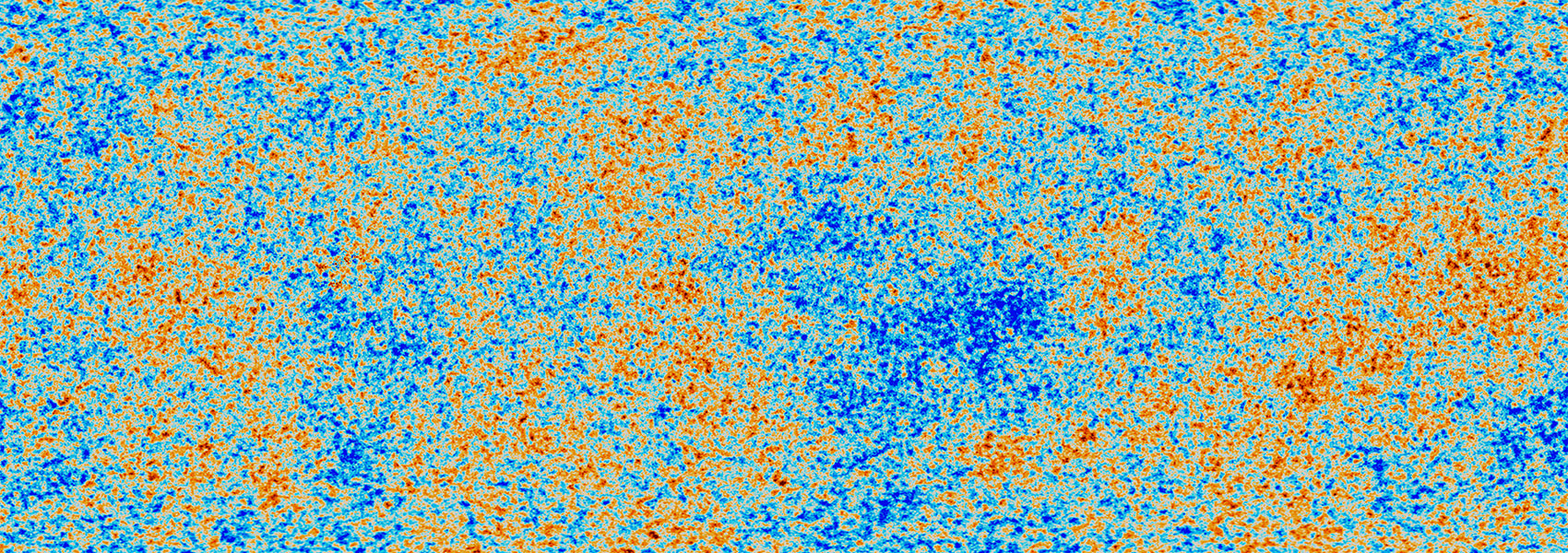# TOI-712: A System of Adolescent Mini-Neptunes Extending to the Habitable Zone

August 2022 • 2022AJ....164...71V

Authors • Vach, Sydney • Quinn, Samuel N. • Vanderburg, Andrew • Kane, Stephen R. • Collins, Karen A. • Kraus, Adam L. • Zhou, George • Medina, Amber A. • Schwarz, Richard P. • Collins, Kevin I. • Conti, Dennis M. • Stockdale, Chris • Massey, Bob • Suarez, Olga • Guillot, Tristan • Mekarnia, Djamel • Abe, Lyu • Dransfield, Georgina • Crouzet, Nicolas • Triaud, Amaury H. M. J. • Schmider, François-Xavier • Agabi, Abelkrim • Buttu, Marco • Hellier, Coel • Furlan, Elise • Gnilka, Crystal L. • Howell, Steve B. • Ziegler, Carl • Briceño, César • Law, Nicholas • Mann, Andrew W. • Rudat, Alexander • Colon, Knicole D. • Rose, Mark E. • Kunimoto, Michelle • Günther, Maximilian N. • Charbonneau, David • Ciardi, David R. • Ricker, George R. • Vanderspek, Roland K. • Latham, David W. • Seager, Sara • Winn, Joshua N. • Jenkins, Jon M.

Abstract • As an all-sky survey, NASA's Transiting Exoplanet Survey Satellite (TESS) mission is able to detect the brightest and rarest types of transiting planetary systems, including young planets that enable study of the evolutionary processes that occur within the first billion years. Here we report the discovery of a young, multiplanet system orbiting the bright K4.5V star, TOI-712 (V = 10.838, ${M}_{\star }={0.733}_{-0.025}^{+0.026}\,{M}_{\odot }$ , R = 0.674 ± 0.016 R , ${T}_{\mathrm{eff}}={4622}_{-60}^{+61}$ K). From the TESS light curve, we measure a rotation period of 12.48 days and derive an age between about 500 Myr and 1.1 Gyr. The photometric observations reveal three transiting mini-Neptunes ( ${R}_{b}={2.049}_{-0.080}^{+0.120}\,{R}_{\oplus }$ , ${R}_{c}={2.701}_{-0.082}^{+0.092}\,{R}_{\oplus }$ , ${R}_{d}={2.474}_{-0.082}^{+0.090}\,{R}_{\oplus }$ ), with orbital periods of P b = 9.531 days, P c = 51.699 days, and P d = 84.839 days. After modeling the three-planet system, an additional Earth-sized candidate is identified, TOI-712.05 (P = 4.32 days, R P = 0.81 ± 0.11 R ). We calculate that the habitable zone falls between 0.339 and 0.844 au (82.7 and 325.3 days), placing TOI-712 d near its inner edge. Among planetary systems harboring temperate planets, TOI-712 (T = 9.9) stands out as a relatively young star bright enough to motivate further characterization.